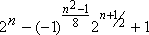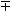# Gaussian Mersenne

Recall that the Mersenne primes are the primes of the form 2n-1. There are no primes of the form bn-1 for any other positive integer b because these numbers are all divisible by b-1. This is a problem because b-1 is not a unit (that is, it is not +1 or -1).

But what if we switch to the Gaussian integers, are there any Gaussian Mersenne primes? That is, are there any Gaussian primes of the form bn-1? If so, then b-1 would have to be a unit. The Gaussian integers have four units: 1, -1, i, and -i. If b-1 is 1, then we get the usual Mersenne primes. If b-1 = -1, then bn-1 is -1, so there are no primes here! Finally if b-1 = ± i, then we get the conjugate pairs of numbers (1 ± i) n-1 with normsand these can be prime!

It is easy to show that a Gaussian integer a+bi is a Gaussian prime if and only if its norm

N(a + bi) = a2+b2

is prime or b=0 and a is a prime congruent to 3 (mod 4). For example, the prime factors of two are 1+i and 1-i, both of which have norm 2. So we have the following result:

Theorem. (1 ± i)n - 1 is Gaussian Mersenne prime if and only if n is 2, or n is odd and the normis a rational prime.

These norms have been repeatedly studied as part of the effort to factor 2n± 1 because they occur as factors in Aurifeuillian factorization

24m-2 + 1 = (22m-1 + 2m + 1) (22m-1 - 2m + 1).

So the first 23 examples of Gaussian Mersennes norms can be found in table 2LM of [BLSTW88], 21 of these were known by the early 1960's. These correspond to the Gaussian Mersenne primes (1 ± i)n-1 for the following values of n:

2, 3, 5, 7, 11, 19, 29, 47, 73, 79, 113, 151, 157, 163, 167, 239, 241, 283, 353, 367, 379, 457, 997.

Much earlier, the mathematician Landry devoted a good part of his life to factoring 2n+1 and finally found the factorization of 258+1 in 1869 (so he was essentially the first to find the Gaussian Mersenne with n=29). Just ten years later, Aurifeuille found the above factorization, which would have made Landry's massive effort trivial [KR98, p. 37]! In all the Cunningham project's papers and books, beginning with [CW25], these Gaussian Mersenne norms have assumed a major role.

In 1961, R. Spira defined the notion a sum of divisor function in the ring of Gaussian integers [Spira61]. He used this to define the Gaussian Mersenne primes as the primes of the form

-i((1+i)k-1)

(associates of the term we define to be a Gaussian Mersenne). W. L. McDaniel [McDaniel73] showed these numbers share many properties with the original Mersenne primes and perfect numbers. In 1976, Hausman and Shapiro started with a more natural definition of perfect numbers (ideals) [HS76].

Mike Oakes, who apparantly originated the approach we used above in the early 1970's, has recently extended the list of known Gaussian Mersennes dramatically. We now know (1 ± i)n-1 is prime for the following values of n:

2, 3, 5, 7, 11, 19, 29, 47, 73, 79, 113, 151, 157, 163, 167, 239, 241, 283, 353, 367, 379, 457, 997, 1367, 3041, 10141, 14699, 27529, 49207, 77291, 85237, 106693, 160423 and 203789.

Gaussian Mersennes share many properties with the regular Mersennes and Oakes suggests they occur with the same density.

Related pages (outside of this work)

References:

BLS75
J. Brillhart, D. H. Lehmer and J. L. Selfridge, "New primality criteria and factorizations of 2m ± 1," Math. Comp., 29 (1975) 620--647.  MR 52:5546 [The article for the classical (n2 -1) primality tests. Table errata in [Brillhart1982]]
BLSTW88
J. Brillhart, D. H. Lehmer, J. L. Selfridge, B. Tuckerman and S. S. Wagstaff, Jr., Factorizations of bn ± 1, b=2,3,5,6,7,10,12 up to high powers, Amer. Math. Soc., 1988.  Providence RI, pp. xcvi+236, ISBN 0-8218-5078-4. MR 90d:11009 (Annotation available)
CW25
A. J. C. Cunningham and H. J. Woodall, Factorizations of yn1, y = 2, 3, 5, 6, 7, 10, 11, 12 up to high powers (n), Hodgson, London, 1925.
HS76
M. Hausmann and H. Shapiro, "Perfect ideals over the gaussian integers," Comm. Pure Appl. Math., 29:3 (1976) 323--341.  MR 54:12704
KR98a
R. Kumanduri and C. Romero, Number theory with computer applications, Prentice Hall, 1998.  Upper Saddle River, New Jersey,
McDaniel73
W. McDaniel, "Perfect Gaussian integers," Acta. Arith., 25 (1973/74) 137--144.  MR 48:11034
Spira61
R. Spira, "The complex sum of divisors," Amer. Math. Monthly, 68 (1961) 120--124.  MR 26:6101